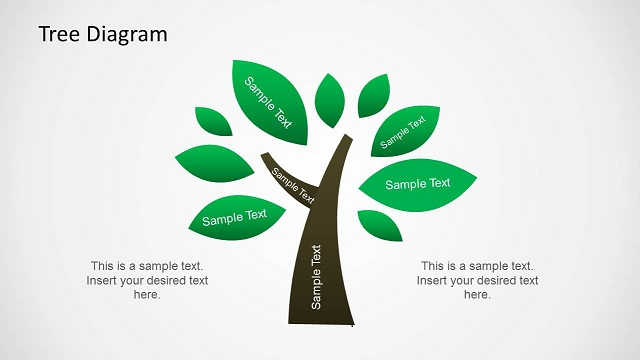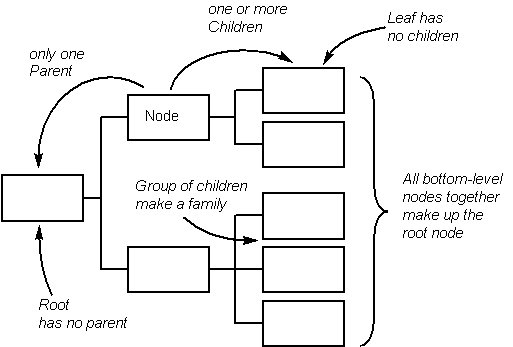# Tree diagram:

Tree diagram is simply a way of representing a sequence of events. Tree diagrams are particularly useful in probability since they record all possible outcomes in a clear and uncomplicated manner.

Example: Let's take a look at a simple example, flipping a coin and then rolling a die. We might want to know the probability of getting a Head and a 4.

If we wanted, we could list all the possible outcomes: (H,1) (H,2) (H,3) (H,4) (H,5) (H,6) (T,1) (T,2) (T,3) (T,4) (T,5) (T,6) Probability of getting a Head and a 4: P(H,4) = 1/12# What's it for?

The Tree Diagram is a deceptively simple tool that can be used in many analysis situation. It can be used to find problem causes in the same way as a Cause-Effect Diagram or be used to find an appropriate solution. It can also be used in design or reverse-design (eg. 'tear-down' of a competitive product), to record how assemblies and sub-assemblies combine to make a complete product. Another use is in planning, to break down a project into manageable tasks. It can be used in a similar way when designing processes, breaking down major activities.

# How does it work?

Many of the problems we face seem large and difficult, with many half-hidden components. The Tree Diagram is a simple method of breaking down such problems into a hierarchy of parts. It works by asking consistent questions of each component, such as 'What parts make up this element?'. The diagram that is created shows clearly how each part relates to another. The names used for parts of the diagram echo both the tree theme and the fact that an early use was in plotting family trees. The other name that is commonly used is more mathematical in origin; the 'node' is a single item on the tree.# How to do it?

Creating a tree is a fairly obvious task, although there are a few tricks described below that can help you on your way.

• As with most tools, start by being clear about the objective, what is in the problem and what is not. For example, a family tree may explicitly exclude or include illegitimate children.
• Define the top-level, 'root' question and place it centre-left (or centre-top, if you prefer). Nodes in the tree can be written directly onto a single sheet of paper, onto thin cards or on adhesive memo notes. The advantage of the latter two methods is that you can easily change your mind and the order of the cards.
• Define the process for breaking down each parent node into a child node. This is typically done by asking the same question, such as, 'Why does this happen?'. A secondary question may also be useful, to help determine when a family is complete. For example, 'Do these children nodes, taken together, completely represent the parent node?'.
• Consistently apply the questions from step 3 to each node to break these down and find their children. Another question is also useful at this stage, to find out when to stop breaking down nodes. For example, when creating a plan, you might ask, 'Can this task be completed in less than a week?'. If the answer is 'yes', then you do not break it down further.
• Review the completed tree and use it for its target purpose.